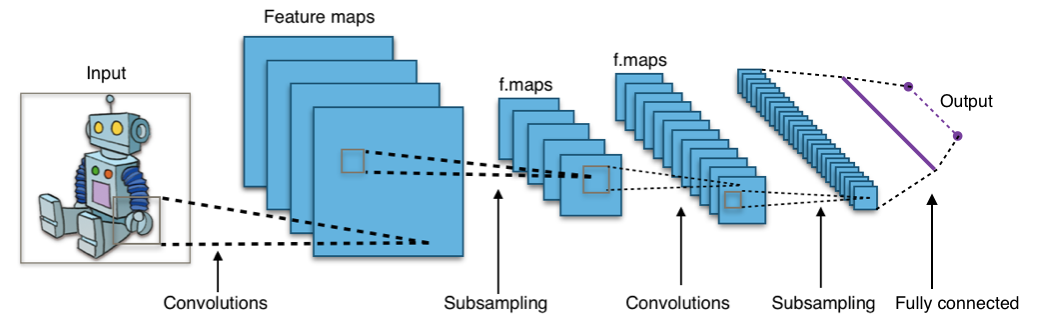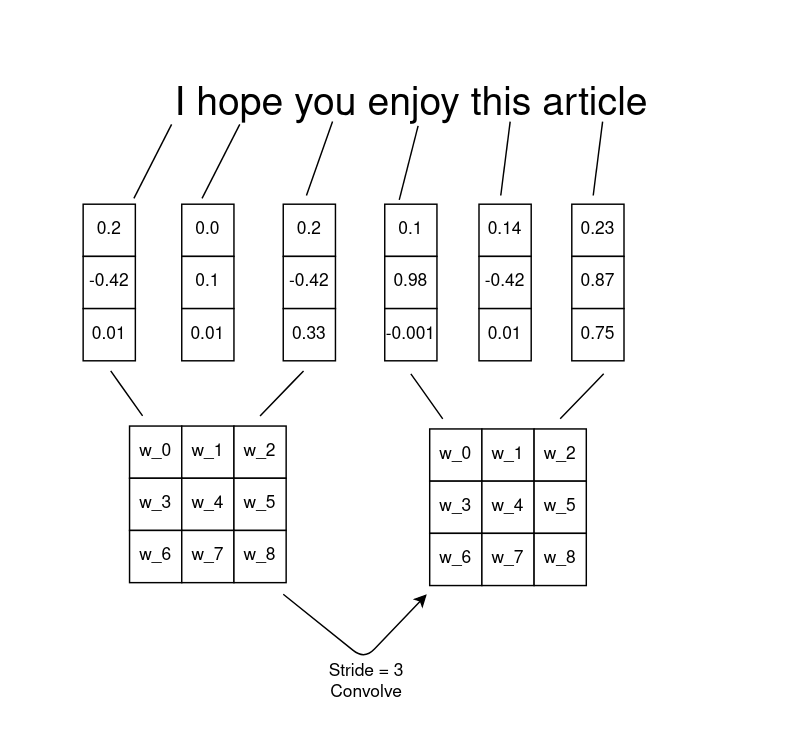# NLP with CNNsIn this article, I will try to explain the important terminology regarding CNNs from a natural language processing perspective, a short Keras implementation with code explanations will also be provided.

Convolutional neural networks (CNNs) are the most widely used deep learning architectures in image processing and image recognition. Given their supremacy in the field of vision, it’s only natural that implementations on different fields of machine learning would be tried. In this article, I will try to explain the important terminology regarding CNNs from a natural language processing perspective, a short Keras implementation with code explanations will also be provided.

The concept of sliding or convolving a pre-determined window of data is the central idea behind why CNNs are named the way they are. An illustration of this concept is as below.Image by author

The first thing to notice here is the method by which each word(token) is represented as 3-dimensional word vectors. A weight matrix of 3x3 is then slid horizontally across the sentence by one step(also known as stride) capturing three words at a time. This weight matrix is called a filter; each filter is also composed of an activation function, similar to those used in feed-forward neural networks. Due to some mathematical properties, the activation function ReLU (rectified linear unit) is mostly used in CNNs and deep neural nets. Going back to image classification, the general intuition behind these filters is that, each filter can detect different features of an image, the deeper the filter, the more likely it will capture more complex details, as an example, the very first filters in your Convnet will detect simple features such as edges and lines, but the features at the very back might be able to detect certain animal types. All this is done without hardcoding any of the filters. Backpropagation will ensure that the weights of these filters are learned from the data.

The next important step is to calculate the output(convolved feature). For the example, below we will consider a 55 image and a 33 filter (when dealing with CNNs you will mostly work with square matrices) the output layer is calculated by summing over the element-wise multiplication as each filter slides over the window of data one stride at a time each pixel is multiplied by its corresponding weight in the filter. The example below illustrates how the first cell in the output layer is calculated; the red numbers in the image represent the weights in the filter.

## Demystifying AI, Machine Learning, and Deep Learning

Demystifying AI, Machine Learning, and Deep Learning. Learn about AI, machine learning, supervised learning, unsupervised learning, classification, decision trees, clustering, deep learning, and algorithms.

## Why Deep Learning Ensembles Outperform Bayesian Neural Networks

Don’t they do the same thing? Why Deep Learning Ensembles Outperform Bayesian Neural Networks

## Should we use Machine Learning or Deep Learning?

To recap the differences between the two: Machine learning uses algorithms to parse data, learn from that data, and make informed decisions based on what it has learned. Deep learning structures algorithms in layers to create an "artificial neural network” that can learn and make intelligent decisions on its own.

## Cheat Sheets for AI, Neural Networks, Machine Learning, Deep Learning & Big Data

Cheat Sheets for AI, Neural Networks, Machine Learning, Deep Learning & Big Data

## How To Get Started With Machine Learning With The Right Mindset

You got intrigued by the machine learning world and wanted to get started as soon as possible, read all the articles, watched all the videos, but still isn’t sure about where to start, welcome to the club.